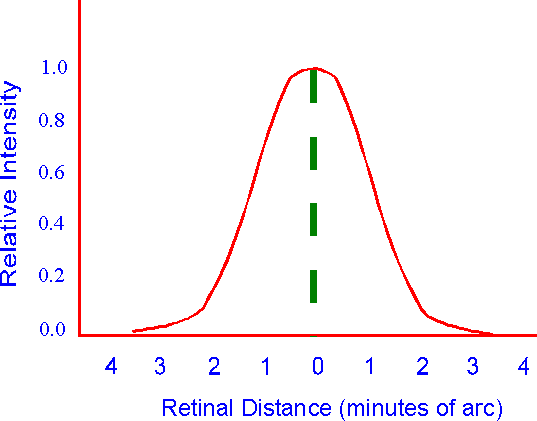### Point Spread FunctionConsider a very small point of light. If the visual system had perfect optics the image of this point on the retina would be identical to the original point of light. So if the relative intensity of this point of light were plotted as a function of distance, on the retina, such a plot would look like the dashed, vertical, green line. However, the eye's optics are not perfect so the relative intensity of the point of light is distributed across the retina as shown by the red curve. This curve is called the "point spread function" (PSF).

More On Spread Functions